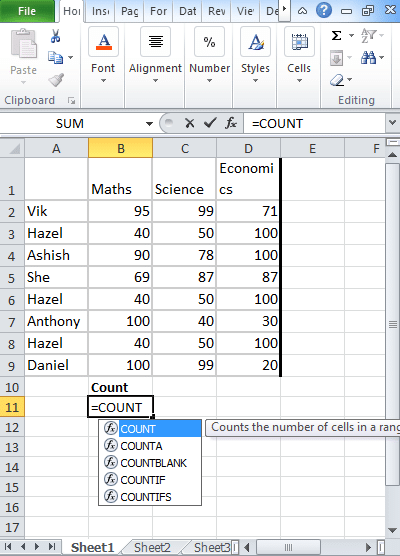To advertise with us contact on Whatsapp: +923041280395 For guest post email at: [email protected]

# Excel Count, CountA, CountBlank, CountIf, CountIfs functionsThe COUNT function will count the total number of cells containing the total number of cells within the list of arguments. The excel count function will return the total number of entries that come under the range or array of numbers.

## Different Types of Excel Count

There are four main count functions in excel listed below:

1. Count
2. CountA
3. CountBlank
4. CountIF
5. CountIFs

Let try doing a hands-on exercise the time you read this tutorial. Open excel file online or alternatively use the one attached here (Count functions.xls). Notice that there is a table with student and subject’s names. The table list down student’s marks in respective subjects.

Count function: It is used to give the total number of cells which contains the numbers.

In cell B11, start typing “=count(” and then select the range of data (in this case from B2 to D9, followed by closing ). COUNT function will give you no. of excel cells where there is any numeric value. In this case, all numbers are in range B2 to D9 making it a total of 24.

CountA function: CountA function will give you the total number of nonblank values.

In cell C11, type “=CountA(” and then select the range of data. In our case, the total no. of values is 35. All cells have data barring B1, B9, C2, C9, D2, and D9)

CountBlank function: CountBlank function will give you total no. of blank cells in the excel data set.

IN cell D12, type “= CountBlank(” and then select the range of data amongst which you want to find blanks. Here, you might have guessed it right. The count blank function will show the result as 5.

Notice if I sum CountBlank and CountA, the sum is total no. of cells in the data range. See, there are 10 rows and 4 columns in the highlighted table. This makes it a total of 4*10 = 40 cells.

CountIF function: The CountIf function will count the total number of cells depending on the condition applied.

IN cell E12, type “=CountIF(“. The first parameter is the criteria range, so go ahead and select the cells from A1 to D10 by using your mouse or alternatively, type A1: D10.

Type, and the second parameter which is the matching condition. Type “Hazel”, close the parentheses ) and press enter. Syntax: “=CountIf(A1:D10, “Hazel”)

You will see that Excel gives you an output of 3. There is exactly three times word Hazel appears in our selected data.

CountIFs function: The CountIfs function counts the total number of cells after applying different conditions.

Let’s try to find out the occurrence where marks are between 25 and 35. In cell E12, type =CountIFS(. Select the range first as it is with all count functions. Type in semicolon <35, select same data range again, type second criteria i.e. >25 and close the bracket. Thus, your formula will look like =CountIFS(B2:D9,”<35″, B2:D9,”>25″).

Look at the data set in the excel. There is one cell D7 where Anthony has scored 30 marks in economics. Thus, Excel returns a value of 1.

Hope the above steps helped you become the master of count functions. Try experimenting with the above formulas – think of any criteria and start checking if your count function is returning the desired results.